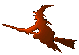Hex  1.0 Hydrogen-electron collision solver
Bspline Class Reference

B-spline environment. More...

#include <bspline.h>

## Public Member Functions

Bspline (int order, rArrayView const &rknots, double th, rArrayView const &cknots)
Constructor. More...

Complex bspline (int i, int iknot, int k, Complex r) const
Evaluate B-spline. More...

Complex dspline (int i, int iknot, int k, Complex r) const
Evaluate derivative of a B-spline. More...

void B (int i, int iknot, int n, const Complex *x, Complex *y) const
B-spline. More...

void dB (int i, int iknot, int n, const Complex *x, Complex *y) const
Derivative of a B-spline. More...

Complex rotate (double r) const
Apply the ECS transformation. More...

double unrotate (Complex z) const
Apply the inverse ECS transformation. More...

cArray zip (const cArrayView coeff, const rArrayView grid) const
Zip 1D expansion. More...

cArray zip (const cArrayView coeff, const rArrayView xgrid, const rArrayView ygrid) const
Zip 2D expansion. More...

void writeVTK (std::ofstream &out, const cArrayView coeff, const rArrayView xgrid, const rArrayView ygrid) const
Zip 2D expansion to VTK. More...

int knot (Complex x) const
Get knot index for coordinate. More...

Complex eval (const cArrayView coeff, double x) const
Evaluate 1D B-spline expansion. More...

Complex eval (const cArrayView coeff, double x, double y) const
Evaluate 2D B-spline expansion. More...

Complex const & t (int i) const
B-spline knot sequence. More...

int Nspline () const
Number of B-splines. More...

int Nknot () const
Number of knots. More...

int Nreknot () const
Number of real knots. More...

int order () const
B-spline order. More...

double R0 () const
End of real grid. More...

double Rmax () const
End of complex grid (real, unrotated). More...

double ECStheta () const
ECS rotation angle. More...

rArray const & rknots () const
real knots More...

rArray const & cknots () const
complex knots More...

## Detailed Description

This class is used to manage B-spline computations within the context of the exterior complex scaling. That is, it allows (upon construction) specification of the real and complex knot sequences

$t_1^{R}, t_2^{R}, ..., t_{\mathrm{Nreknot}}^{R} \ ,$

$t_{\mathrm{Nreknot}+1}^{C}, ..., t_{\mathrm{Nknot}}^{C} \ ,$

which then serve as the knot data of the B-spline set. See bspline and dspline on the definition formulae.

## Constructor & Destructor Documentation

 Bspline::Bspline ( int order, rArrayView const & rknots, double th, rArrayView const & cknots )

Setup the knot sequence, which will consist of two parts.

Parameters
 order B-spline order. rknots Real knot array (usually including R₀). th ECS angle in radians. cknots To-be-complex knot array (including R₀ and Rmax).

## Member Function Documentation

 void Bspline::B ( int i, int iknot, int n, const Complex * x, Complex * y ) const

Vectorized interface to the bspline function. For parameters description see bspline.

 Complex Bspline::bspline ( int i, int iknot, int k, Complex r ) const

Function evaluates a B-spline in one point. The recursive Cox de Boor formula is used:

$B_i^{(0)}(x) = \cases { 1 & t_i \le x \le t_{i+1} \cr 0 & \mbox{otherwise} }\ ,$

$B_i^{(k)}(x) = \frac{x - t_i}{t_{i+k-1} - t_i} B_i^{(k-1)}(x) + \frac{t_{i+k} - x}{t_{i+k} - t_{i+1}} B_{i+1}^{(k-1)}(x) \ .$

Parameters
 i Index of the B-spline. iknot Index of the left knot. k B-spline order. r Coordinate (independent variable).
 rArray const& Bspline::cknots ( ) const
inline
 void Bspline::dB ( int i, int iknot, int n, const Complex * x, Complex * y ) const

Vectorized interface to the dspline function. For parameters description see bspline.

 Complex Bspline::dspline ( int i, int iknot, int k, Complex r ) const

This function evaluated the derivative of a B-spline, using the formula

Parameters
 i Index of the B-spline. iknot Index of the left knot. k B-spline order. r Coordinate (independent variable).
 double Bspline::ECStheta ( ) const
inline
 Complex Bspline::eval ( const cArrayView coeff, double x ) const

Evaluates a B-spline expansion in a single point x. This function is faster than calling

* Complex z = zip (coeff, rArray({x}));
*

though the results are the same.

 Complex Bspline::eval ( const cArrayView coeff, double x, double y ) const

Evaluates a double B-spline expansion in a single point (x,y). This function is faster than calling

* Complex z = zip (coeff, rArray({x}), rArray({y}));
*

though the results are the same.

 int Bspline::knot ( Complex x ) const

Finds knot (interval $$\left< t_i,t_{i+1} \right>$$) for 'x'.

Parameters
 x Complex coordinate.
 int Bspline::Nknot ( ) const
inline
 int Bspline::Nreknot ( ) const
inline
 int Bspline::Nspline ( ) const
inline
 int Bspline::order ( ) const
inline
 double Bspline::R0 ( ) const
inline
 rArray const& Bspline::rknots ( ) const
inline
 double Bspline::Rmax ( ) const
inline
 Complex Bspline::rotate ( double r ) const
inline
Parameters
 r Real coordinate.
 Complex const& Bspline::t ( int i ) const
inline
 double Bspline::unrotate ( Complex z ) const
inline
Parameters
 z Complex coordinate.
 void Bspline::writeVTK ( std::ofstream & out, const cArrayView coeff, const rArrayView xgrid, const rArrayView ygrid ) const
 cArray Bspline::zip ( const cArrayView coeff, const rArrayView grid ) const

Evaluate 1D function given as a B-spline expansion over a grid.

Parameters
 coeff Expansion coefficients. grid Evaluation points (unrotated). They are assumed to be sorted. The length of coeff must be at least equal to the spline count and it is these first Nspline coefficients that are used in evaluation.
 cArray Bspline::zip ( const cArrayView coeff, const rArrayView xgrid, const rArrayView ygrid ) const

Evaluate 2D function given as a B-spline expansion over a carthesian product of two 1D grids.

Parameters
 coeff Expansion coefficients. xgrid Evaluation points at x-axis (unrotated). They are assumed to be sorted. ygrid Evaluation points at y-axis (unrotated). They are assumed to be sorted. The length of coeff must be at least equal to the spline count squared and it is these first Nspline**2 coefficients that are used in evaluation.

The documentation for this class was generated from the following files: